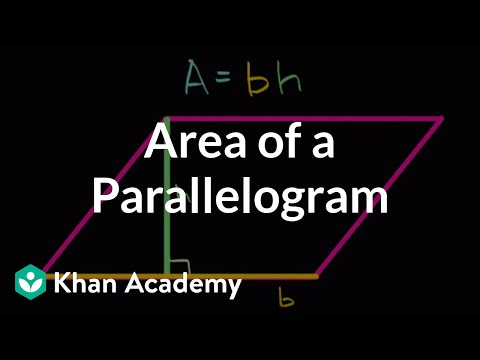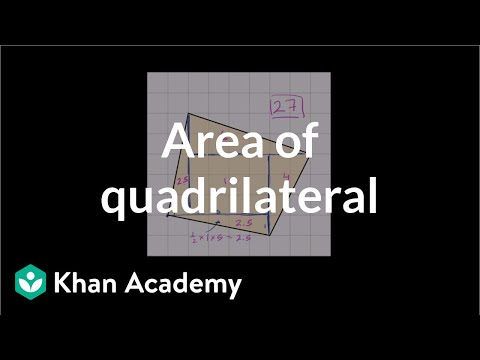# Measuring area with partial unit squares (Full video)

Description: Lindsay finds the area of a shape by counting whole and partial unit squares. Each square on the a grid is unit square with an area of one square centimeter. How many square centimeters does the quadrilateral cover? Here's one square centimeter the quadrilateral covers, and I can keep counting like that, all of the square centimeters that I can see.

### Other videos you might be interested in### Area of a parallelogram (Full video)